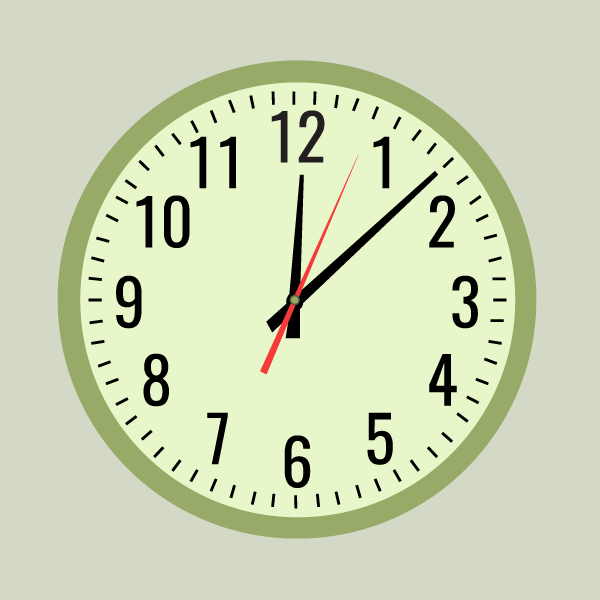# Getting Date and Time in Node.js

nodejs
Published on June 29, 2019#### Use-Cases of this Tutorial

• Know how to get the current date, month, year, hour, minutes & seconds in Node.js
• Know how to get the current date-time formatted as YYYY-MM-DD hh:mm:ss, YYYY-MM-DD, DD-MM-YYYY etc
• Know how to get the current timestamp
• Know how to get date-time from a given timestamp

In Node.js date and time are handled with the Javascript Date object. It is loaded by default and requires no import of modules.

#### Getting Current Date and Time as YYYY-MM-DD hh:mm:ss

The current date and time can be fetched by first creating a new Date object. Thereafter methods of the object can be called to get the date and time values.

``````// new Date object
let date_ob = new Date();
``````
• getDate : returns the day of the month (1-31)
• getMonth : returns the month as an integer (0-11). Note that January is represented as 0 and December as 11.
• getFullYear : returns the year in 4-digit format
• getHours: returns the hour of the day in 24-hour format (0-23)
• getMinutes: returns the minute (0-59)
• getSeconds: returns the seconds (0-59)

Using the above methods one can contruct the date and time as YYYY-MM-DD hh:mm:ss format, or rather to any format. Note that because some methods return single-digit values, we will need to append 0 before them (for example "5" becomes "05").

``````let date_ob = new Date();

// current date
// adjust 0 before single digit date
let date = ("0" + date_ob.getDate()).slice(-2);

// current month
let month = ("0" + (date_ob.getMonth() + 1)).slice(-2);

// current year
let year = date_ob.getFullYear();

// current hours
let hours = date_ob.getHours();

// current minutes
let minutes = date_ob.getMinutes();

// current seconds
let seconds = date_ob.getSeconds();

// prints date in YYYY-MM-DD format
console.log(year + "-" + month + "-" + date);

// prints date & time in YYYY-MM-DD HH:MM:SS format
console.log(year + "-" + month + "-" + date + " " + hours + ":" + minutes + ":" + seconds);

// prints time in HH:MM format
console.log(hours + ":" + minutes);
``````

#### Getting Current Timestamp

To get the timestamp Date.now() method can be called. Note that this method returns the timestamp in milliseconds. To get the timestamp as seconds we can divide it by 1000.

``````let ts = Date.now();

// timestamp in milliseconds
console.log(ts);

// timestamp in seconds
console.log(Math.floor(ts/1000));
``````

#### Getting Date and Time from Timestamp

In order to get the date and time values from a given timestamp, the timestamp is passed as a parameter to the Date constructor.

Then the above mentioned methods can be called to get a date and time string.

Note that Javascript timestamps are specified as milliseconds, so if the given timestamp is in seconds you will need to convert the same to milliseconds by multiplying with 1000.

``````// current timestamp in milliseconds
let ts = Date.now();

let date_ob = new Date(ts);
let date = date_ob.getDate();
let month = date_ob.getMonth() + 1;
let year = date_ob.getFullYear();

// prints date & time in YYYY-MM-DD format
console.log(year + "-" + month + "-" + date);
``````